A Comment on Sample Size Calculation for Analysis of Covariance in Parallel Arm Studies | OMICS International
Journal of Biometrics & Biostatistics

Like us on:

# A Comment on Sample Size Calculation for Analysis of Covariance in Parallel Arm Studies

Guogen Shan1* and Changxing Ma2

1Epidemiology and Biostatistics Program, Department of Environmental and Occupational Health, School of Community Health Sciences, University of Nevada Las Vegas, Las Vegas, NV 89154, USA

2Department of Biostatistics, University at Buffalo, Buffalo, NY 14214, USA

*Corresponding Author:
Guogen Shan
Epidemiology and Biostatistics Program, Department of Environmental and Occupational Health
School of Community Health Sciences
University of Nevada Las Vegas, Las Vegas, NV 89154, USA
Tel: 702-895-4413
Fax: 702-895-5184
E-mail: [email protected]

Received date: December 17, 2013; Accepted date: January 09, 2014; Published date: January 16, 2014

Citation: Shan G, Ma C (2014) A Comment on Sample Size Calculation for Analysis of Covariance in Parallel Arm Studies. J Biomet Biostat 5: 184. doi: 10.4172/2155-6180.1000184

Copyright: © 2014 Shan G, et al. This is an open-access article distributed under the terms of the Creative Commons Attribution License, which permits unrestricted use, distribution, and reproduction in any medium, provided the original author and source are are credited.

Visit for more related articles at Journal of Biometrics & Biostatistics

#### Abstract

We compare two sample size calculation approaches for analysis of covariance with one covariate. Exact simulation studies are conducted to compare the sample size calculation based on an approach by Borm et al. (2007) (referred to as the B approach) and an exact approach (referred to as the F approach). Although the B approach and the F approach have similar performance when the correlation coefficient is small, the F approach generally has a more accurate sample size calculation as compared to the B approach. Therefore, the F approach for sample size calculation is generally recommended for use in practice.

#### Keywords

Analysis of covariance; F distribution; Sample size; Power

#### Introduction

Randomized clinical trials are commonly used to confirm the efficacy of a new treatment. There are several advantages for using randomization in clinical trials, such as selection bias reduction and increased comparability among groups with potential confounding factors . Balanced studies are often conducted to maximize the power of a study for a given total sample size.

Sample size calculation plays a very important role in clinical trials. It has been studied for many years and achieved significant progress [2-4]. As far as we know, sample size calculation approaches for analysis of covariance (ANCOVA) are very limited. Recently, Borm et al.  proposed a simple sample size calculation closed form for ANCOVA with one covariate which is considered as a baseline of the response outcome. Based on the sample size from a two sample t-test and the correlation between response outcomes and covariate values, they show that this formula has accurate sample size calculation. The other method is based on a ratio of mean squares [6,7] where the null distribution follows a F distribution and the alternative is a non-central F distribution. There is no systematic comparison between these two approaches.

We reviewed two existing sample size calculation approaches for ANCOVA with one covariate in Section 2. In Section 3, we compare the two approaches using exact simulation studies and an example from a randomized study is used to illustrate these two approaches. Section 4 is given to discussion.

#### Methods

Suppose that Yij be the jth response outcome for the ith group, i=1, 2; j=1,2,…,ni, and Xij be the associated covariate. We consider the first group as the control, and the second group as the treatment group in this article. The covariate can be viewed as the baseline for the output. The regression model for the relationship between Y and X within the ith group is given as

Yij0i1Xijij,

where β0i is the intercept for the ith group, β1 is the common slope for both groups, and εij is the measure error which follows a normal distribution . The mean difference between two groups is the difference between two intercepts.

Borm et al.  proposed a simple sample size calculation for ANCOVA by multiplying the number of subjects for the two sample t-test by a design factor. The factor here is 1−ρ2, where ρ is the correlation coefficient between the outcome and the covariate. Sample size calculation for the two-sample t-test is based on response outcomes. Given a significance level of α, a pre-specified power 1−β, the mean difference between the treatment group and the control of μ2−μ1, and a common standard deviation of response outcome σ, sample size per group is calculated as

n=2σ2(Z1−α/2+Z1−β)2/(μ2−μ1)2,

where Zd is the d−th percentile of a standard normal distribution. Borm et al.  showed that the total sample size for the ANCOVA N=2n(1− ρ2) may not be accurate enough for small sample settings to retain the pre-specified power. They provided some power plots to show that power with this sample size formula is generally smaller than 1−β for small sample settings. For this reason, they proposed

N=2(n + 1)(1−ρ2)

to be used as the sample size by adding one subject for each group in the sample size calculation. They claimed that this sample size is accurate for all sample sizes.

The second method is an exact approach based on a ratio of mean squares,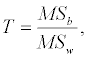where MSb is the mean square between groups, and MSw is the mean square within the group . Under the null hypothesis with no difference between the control and the treatment group, the ratio T follows a central F1,N−3 distribution. Given a significance level of α, the threshold value is Fα, where Pr(F1,N−3 ≥ Fα)=α. Under the alternative, the test statistic follows a non-central F1,N−3,λ, distribution with the non-central parameter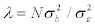, where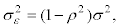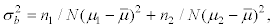and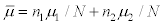is the overall response outcome mean. The power of the study is then expressed as a probability of being greater than or equal to the threshold Fα in the non-central F distribution, Pr(F1,N−3,λ ≥ Fα). The required sample size is determined by increasing the sample size by one each time until the pre-specified power is reached.

Method comparison

We referred to the approach proposed by Borm et al.  as the B approach, and the other based on the F distribution as the F approach. Power is calculated as the percentage of trials with significant p-values using ANCOVA based on 10000 simulations. Calculated power is presented in Table 1 for α=0.05, β=0.2, σ=1, and μ2−μ1=0.5, and Table 2 for α=0.01, β=0.2, σ=1, and μ2−μ1=1. Sample size based on the F approach is calculated using PASS 12 . As can be seen from both tables, the difference between the B approach and the F approach is negligible for small ρ values. The power of the B approach is much lower than the pre-specified power for large ρ values, as shown in Table 2, the power could be as low as 52%. Although the B approach and the F approach have similar performance when ρ is small, the F approach generally has more accurate sample size calculation as compared to the B approach.

ρ Borm approach non-central F distribution
n per group power n per group power
0 64 0.7941 64 0.7965
0.1 64 0.802 64 0.8019
0.2 62 0.7961 62 0.8057
0.3 59 0.8081 59 0.7988
0.4 54 0.803 54 0.7974
0.5 48 0.7945 49 0.8082
0.6 41 0.7957 42 0.7943
0.7 33 0.7836 34 0.7989
0.8 23 0.7758 24 0.8046
0.9 13 0.7842 14 0.8154

Table 1: alpha=0.05, power=0.8, sd=1, diff=0.5.

ρ Borm approach non-central F distribution
n per group power n per group power
0 25 0.7918 26 0.806
0.1 25 0.7891 25 0.7917
0.2 24 0.7874 25 0.8059
0.3 23 0.7966 24 0.8096
0.4 21 0.782 22 0.8037
0.5 19 0.7839 20 0.7996
0.6 16 0.7629 17 0.795
0.7 13 0.7452 14 0.7975
0.8 9 0.6765 11 0.8117
0.9 5 0.5197 7 0.8126

Table 2: alpha=0.01, power=0.8, sd=1, diff=1.

A parallel randomized clinical trial is illustrated for sample size calculation based on the B approach and the F approach. Patients with rheumatoid arthritis are randomized into one of the groups with or without leunomide . The response outcome, the disease activity score, is measured before and after the treatment. The baseline measurement is considered as the covariate in the ANCOVA model. This example is also used by Borm et al. . The standard deviation is estimated as σ=1.2. At a significance level of α=0.01 and 90% power, the sample size calculations based on the B approach to detect a mean difference of μ2−μ1=0.6 are 122, 86, and 46 as total sample sizes for ρ=0.7, 0.8, and 0.9, respectively. It needs total sample sizes of 126, 90, and 50 using the F approach. The sample size from the B approach is less than that from the F approach. The sample size from the B approach may not attain the pre-specified power of the study.

#### Conclusions

The sample size calculation formula proposed by Borm et al.  has a closed form, and it is computationally easy. Power of the study may be lower than the pre-specified power when ρ is large. Power of the F approach is closer to the pre-specified power for all ρ values. The code written in R for the sample size calculation for both methods is available from the first author. In a study with multiple covariates, Borm et al.  recommended using 1−R2 as the design factor. We consider the comparison between this approach and the approach based on the ratio of the mean squares as future work. Another possible future work would be sample size calculation based on exact approaches [11-14].

#### Acknowledgements

Dr. Shan's research is partially supported by a Faculty Opportunity Awards from UNLV.

#### References

Select your language of interest to view the total content in your interested language

### Article Usage

• Total views: 12656
• [From(publication date):
February-2014 - Dec 07, 2019]
• Breakdown by view type
• HTML page views : 8815Can't read the image? click here to refresh